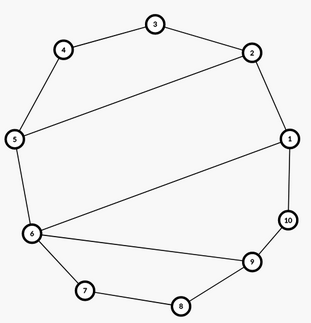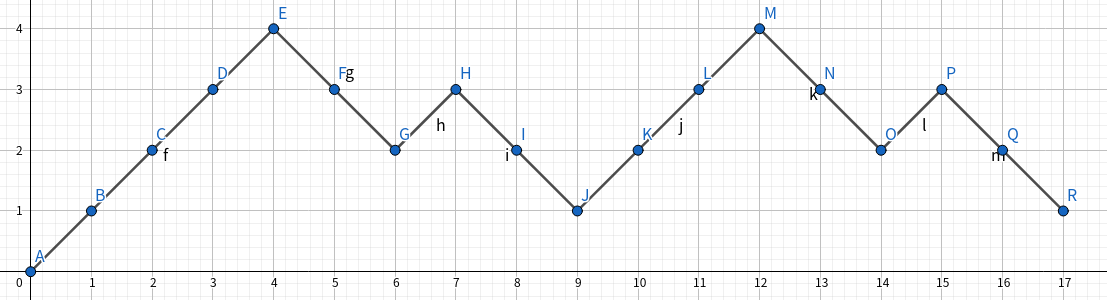### 基础知识

#### 卡特兰数的式子

\begin{aligned} &h(n)=\sum_{i=0}^{n-1}h(i)\cdot h(n-i-1)\\ &h(n)=\frac{h(n-1)\cdot(4n-2)}{n+1}\\ &h(n)=\frac{\binom{2n}{n}}{n+1}=\frac{\binom{2n+1}{n}}{2n+1} \end{aligned}

#### 卡特兰数的例子

• 合法出栈入栈操作序列。
• 合法括号序列。
• 凸多边形划分为三角形的方案数。

### 拓展

#### 广义卡特兰数

$$h(n)=\sum_{S}\prod_{x\in S} h(x)\ [\ |S|=k-1,\sum_{x\in S}x=n-1]$$

$$H(n)=xH^{k-1}(n)+1$$